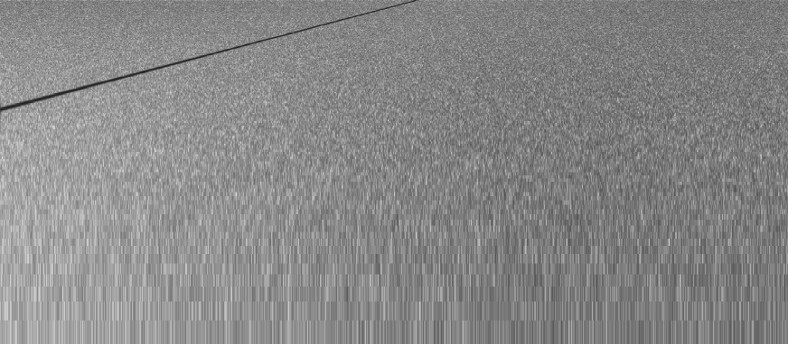# mathr

## Non-uniform sampling

Recently I've been experimenting with different ways to try to improve image quality of fractal renders. The first key step was using jitter, which is adding independent uniform random offsets to the pixel coordinates before calculating the fractal iterations. This converts strong aliased frequencies (like Moiré patterns) into much perceptually acceptable noise with a broad spectrum. The noise can be reduced by averaging many images with different pseudo-random number generator seed values. I used a Wang/Burtle uint32 hash of the pixel coordinates and a subframe index, the quality of which isn't well known, but visually indistinguishable from the cryptographic hash MD5 in my tests.

Unfortunately it's not perfect, artifacts can return to visibility after averaging (which means they were never completely eliminated in the first place, possibly the colouring algorithm computing image-space derivatives of the fractal iteration data might have defeated the jitter in some way). It seems correctly bandlimiting and sampling fractal images is a hard problem, and simpler techniques like supersampling by a large factor with properly filtered downscaling can be better than jitter alone.

The 1D problem seems more tractable. Here you can see spectrum of the audio version, being a sine sweep from 8kHz going up 4 octaves - with uniform sampling it folds over into a descending sweep:With jittered sampling the aliased energy becomes white noise:Unfortunately the noise reduction from averaging isn't great: doubling the number of jittered copies (each section in the image below) reduces the noise level by only 3dB. Uniformly oversampling by only 6x would eliminate aliasing completely for this toy example, but some signals might not have a known upper bandwidth limit.Performance is terrible too, many minutes of CPU time for the few seconds of output. Anyway, here's source code for the audio experiment, you can listen to the output:

// gcc non-uniform_sampling.c -lfftw3 -lm -O3
// time ./a.out > out.raw
// audacity # import out.raw f64 mono 48000Hz

#include <complex.h>
#include <math.h>
#include <stdio.h>
#include <stdlib.h>
#include <string.h>

#include <fftw3.h>

#define PI 3.141592653589793

// sweep parameters
#define HZ 8000
#define OCTAVES 4
#define SPEED 1
#define SAMPLERATE 48000
#define N (OCTAVES * SAMPLERATE / SPEED)

// fft parameters (size and overlap)
#define F 256
#define O 4

// jitter amount
#define SCALE 1

// buffers
double t[N]; // sample time
double f[N]; // sample value
double g[N]; // accumulated data
double h[N]; // normalized data for output

// fft buffers
fftw_complex *fft;
fftw_complex *sig;

// raised cosine window for overlap-add resynthesis
double window(double t)
{
return 0.5 - 0.5 * cos(2 * PI * t);
}

// entrypoint
int main()
{
// initialize
fft = fftw_malloc(sizeof(fftw_complex) * F);
sig = fftw_malloc(sizeof(fftw_complex) * F);
fftw_plan plan = fftw_plan_dft_1d(F, fft, sig, FFTW_BACKWARD, FFTW_MEASURE);
memset(g, 0, sizeof(g));
// average many passes with distinct random seeds
int pass = 0;
for (int PASSES = 0; PASSES < 10; ++PASSES)
{
for (; pass < 1 << PASSES; ++pass)
{
fprintf(stderr, "%d\n", pass);
// sample non-bandlimited signal at non-uniform intervals
for (int i = 0; i < N; ++i)
{
t[i] = i + (rand() / (double) RAND_MAX - 0.5) * SCALE;
f[i] = sin(2 * PI * exp(log(HZ << OCTAVES) * t[i] / N + (1 - t[i] / N) * log(HZ)));
}
for (int start = -F; start < N; start += F / O)
{
// window and DFT
memset(fft, 0, sizeof(fftw_complex) * F);
for (int i = start; i < start + F; ++i)
{
if (0 <= i && i < N)
{
double t0 = (t[i] - start) / F;
double f0 = window(t0) * f[i];
for (int k = 0; k < F; ++k)
{
double w0 = 2 * PI * (k > F/2 ? k - F : k);
double w = - t0 * w0;
fft[k] += f0 * (cos(w) + I * sin(w));
}
}
}
// do IFFT
fftw_execute(plan);
// window and accumulate
for (int i = start; i < start + F; ++i)
{
if (0 <= i && i < N)
{
double t1 = (i - start) / (double) F;
double w = window(t1);
double s = sig[i - start];
g[i] += w * creal(s);
}
}
}
}
// normalize
double grms2 = 0;
for (int i = 0; i < N; ++i)
grms2 += g[i] * g[i];
grms2 /= N;
grms2 = sqrt(grms2);
grms2 *= 4;
for (int i = 0; i < N; ++i)
h[i] = g[i] / grms2;
// output
fwrite(h, sizeof(h), 1, stdout);
fflush(stdout);
}
// cleanup
fftw_destroy_plan(plan);
fftw_free(sig);
fftw_free(fft);
return 0;
}


Reporting this "failed" experiment in the interest of science!